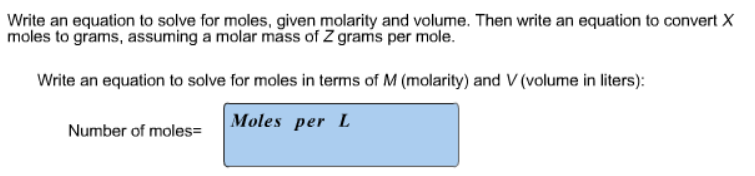# Problem: Write an equation to solve for moles, given molarity and volume. Then write an equation to convert X moles to grams, assuming a molar mass of Z grams per mole. Write an equation to solve for moles in terms of M (molarity) and V (volume in liters):

###### FREE Expert Solution
84% (478 ratings)###### Problem Details

Write an equation to solve for moles, given molarity and volume. Then write an equation to convert X moles to grams, assuming a molar mass of Z grams per mole.

Write an equation to solve for moles in terms of M (molarity) and V (volume in liters):